# 六行python代码的爱心曲线

例如，画出指定区间的一个多项式函数：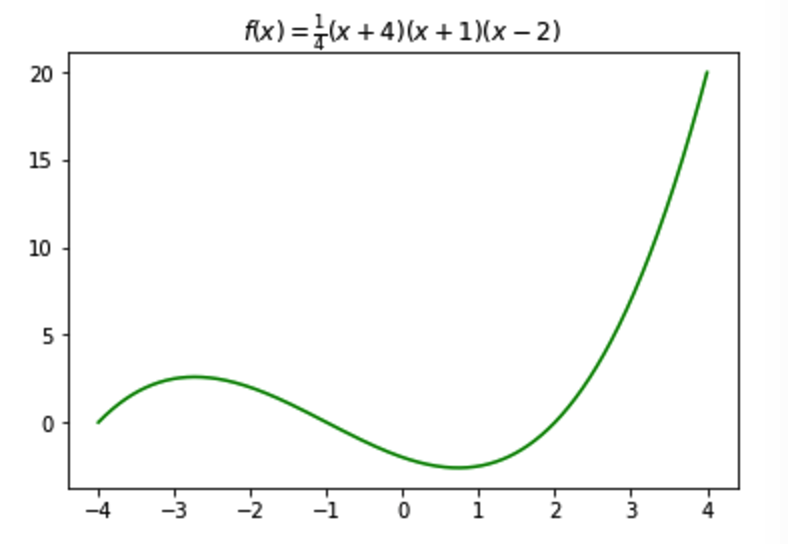Python 代码如下：

import numpy as np
import matplotlib.pyplot as plt
X = np.linspace(-4, 4, 1024)
Y = .25 * (X + 4.) * (X + 1.) * (X - 2.)
plt.title('$f(x)=\\frac{1}{4}(x+4)(x+1)(x-2)$')
plt.plot(X, Y, c = 'g')
plt.show()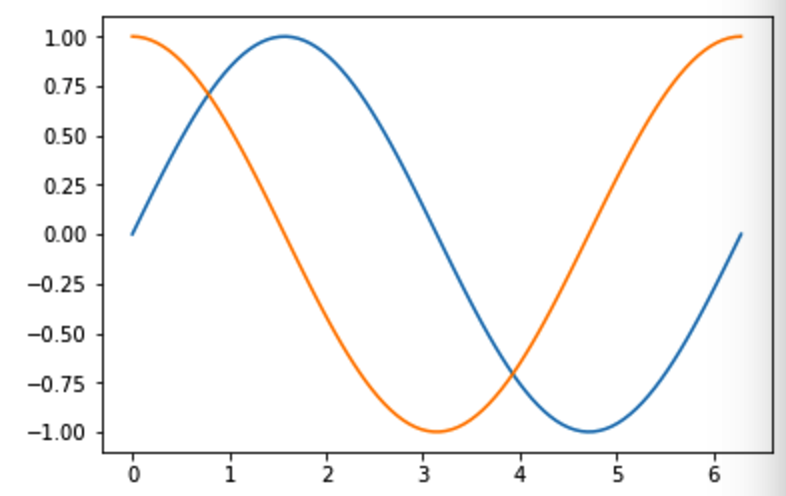Python代码如下：

import numpy as np
import matplotlib.pyplot as plt
X = np.linspace(0, 2 * np.pi, 100)
YSinValues = np.sin(X)
YCosValues = np.cos(X)
plt.plot(X, YSinValues)
plt.plot(X, YCosValues)
plt.show()

Matplotlib 是一个 Python 的 2D绘图库，甚至可以生成出版质量级别的图形。

import numpy as np
import matplotlib.pyplot as plt
def pdf(X, mu, sigma):
a = 1. / (sigma * np.sqrt(2. * np.pi))
b = -1. / (2. * sigma ** 2)
return a * np.exp(b * (X - mu) ** 2)
X = np.linspace(-6, 6, 1000)
for i in range(3):
samples = np.random.standard_normal(10)
mu, sigma = np.mean(samples), np.std(samples)
plt.plot(X, pdf(X, mu, sigma), color = '.66')
plt.plot(X, pdf(X, 0., 1.), color = 'b')
plt.show()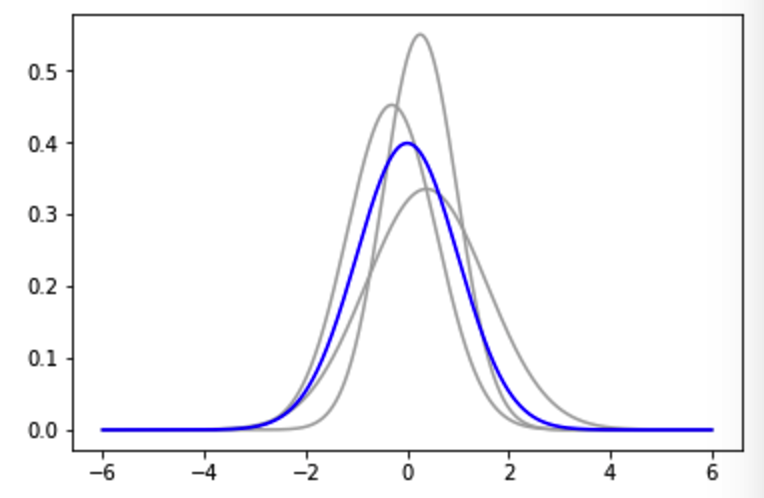300多年前，斯德哥尔摩的街头，落魄的笛卡尔过着乞讨的生活，全部的财产破破烂烂的衣服和随身所带的几本数学书籍。清高的笛卡尔没有开口请求路人施舍，只是默默地低头在纸上写写画画，潜心于他的数学世界。 一个宁静的午后，笛卡尔照例坐在街头的阳光中研究数学问题，身边过往的人群，喧闹的车马队伍，都无法对他造成干扰。

“你在干什么呢？”扭过头，笛卡尔看到一张年轻秀丽的睑庞，一双清澈的眼睛如湛蓝的湖水，楚楚动人，她就是瑞典的小公主，国王最宠爱的女儿克里斯汀。 她蹲下身，拿过笛卡尔的数学书和草稿纸，和他交谈起来。言谈中，他发现，这个小女孩思维敏捷，对数学有着浓厚的兴趣。

几天后，笛卡尔意外地接到通知，国王聘请他做小公主的数学老师。满心疑惑的笛卡尔跟随侍卫一起来到皇宫，他听到了从远处传来的银铃般的笑声。他看到了那天在街头偶遇的女孩子，从此，他当上了公主的数学老师。

公主的数学在笛卡尔的悉心指导下突飞猛进，他们之间也开始变得亲密起来。笛卡尔向她介绍了直角坐标系，代数与几何可以结合起来，也就是日后笛卡尔创立的解析几何学雏形。 在笛卡尔的带领下，克里斯汀走进了奇妙的坐标世界，她对曲线着了迷。每天的形影不离也使他们彼此产生了爱慕之心。

在瑞典这个浪漫的国度里，一段纯粹而美好的爱情悄然萌发。

他们的恋情传到了国王的耳中，国王大怒，下令将笛卡尔处死。在克里斯汀的苦苦哀求下，国王将他放逐回国，公主被软禁在宫中。

当时，欧洲大陆正在流行黑死病。笛卡尔回到法国后不久，便染上重病。在生命进入倒计时的那段日子，他日夜思念的还是街头偶遇的那张温暖笑脸。他每天坚持给她写信，盼望着她的回音。然而，这些信都被国王拦截下来，公主一直没有收到他的任何消息。

在笛卡尔给克里斯汀寄出第十三封信后，他永远地离开了这个世界。此时，被软禁在宫中的小公主依然徘徊在皇宫的走廊里，思念着远方的情人。
这最后一封信上没有写一句话，只有一个方程：r=a(1-sinθ)。
国王以为这个方程里隐藏着两个人的秘密，便把全城的数学家召集到皇宫，但是没有人能解开这个函数式。他不忍看着心爱的女儿每天闷闷不乐，便把这封信给了她。拿到信的克里斯汀欣喜若狂，她立即明白了恋人的意图，找来纸和笔，把图形画了出来，一颗心形图案出现在眼前，克里斯泪流满面，这条曲线就是著名的“心形线”。

国王去世后，克里斯汀继承王位，便立刻派人去法国寻找心上人的下落，收到的却是笛卡尔去世的消息，留下了一个永远的遗憾…… 　　这封享誉世界的另类情书，据说至今还保存在欧洲笛卡尔的纪念馆里。

x=a*(2*cos(t)-cos(2*t))
y=a*(2*sin(t)-sin(2*t))

import numpy as np
import matplotlib.pyplot as plt
a = 1
t = np.linspace(0 , 2 * np.pi, 1024)
X = a*(2*np.cos(t)-np.cos(2*t))
Y = a*(2*np.sin(t)-np.sin(2*t))
plt.plot(Y, X,color='r')
plt.show()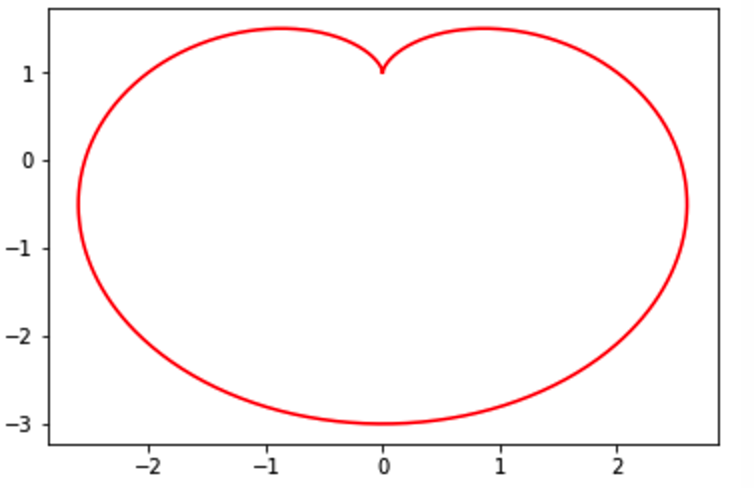import numpy as np
import matplotlib.pyplot as plt
T = np.linspace(0 , 2 * np.pi, 1024)
plt.axes(polar = True)
plt.plot(T, 1. - np.sin(T),color="r")
plt.show()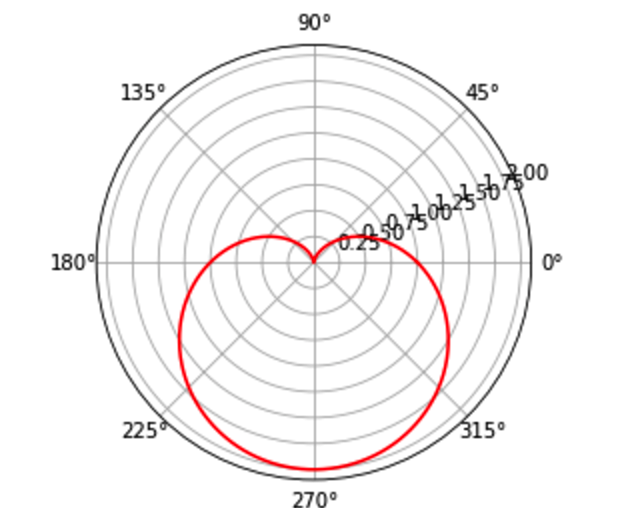import numpy as np
import matplotlib.pyplot as plt

x = np.linspace(-8 , 8, 1024)
y1 = 0.618*np.abs(x) - 0.8* np.sqrt(64-x**2)
y2 = 0.618*np.abs(x) + 0.8* np.sqrt(64-x**2)
plt.plot(x, y1, color = 'r')
plt.plot(x, y2, color = 'r')
plt.show()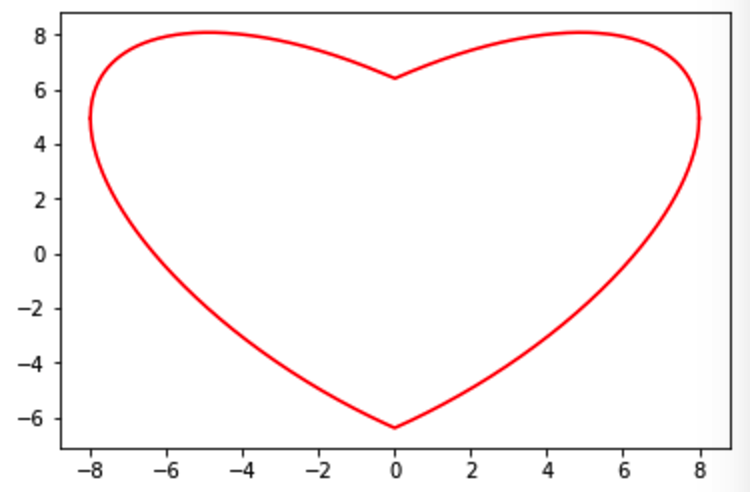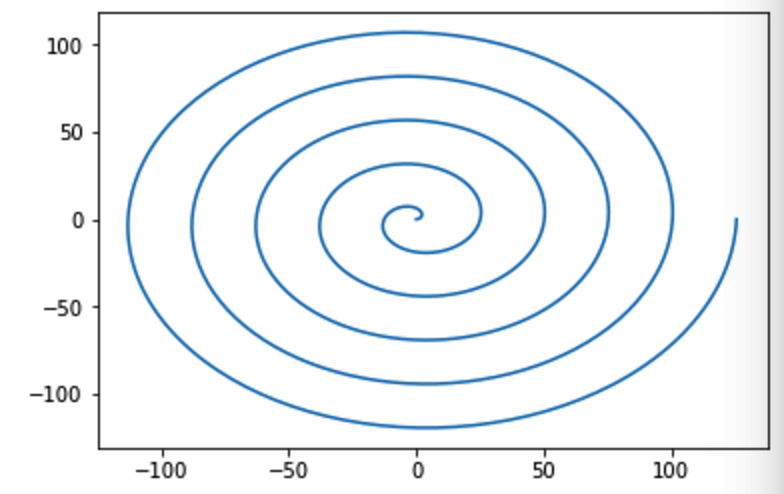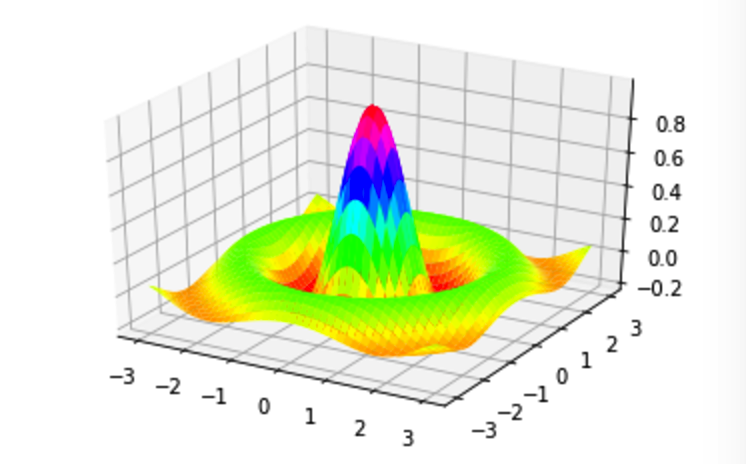##### 参考阅读

http://matplotlib.org/

《Python 数据可视化编程实战》

《Python 数据分析实战》07-23
11-20
02-217070
03-15
08-073万+
12-032万+
11-017428
05-176万+
©️2020 CSDN 皮肤主题: Age of Ai 设计师:meimeiellie